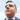# Basic Swift

### Swift variables

#### Types of variables

Swift has 2 types of variables `let` and `var`.

• `let` defines constants. Cannot be changed over time.
• `var` defines any other variable type that can change over time.
``````let secondsInHour = 3600
var milesIDidToday = 24``````

Variables can be defined all in the same line:

``var foo = 2, bar = 3, wow = 5, cat = 7``

#### Type Annotation

Allows to specify what type of variable it is:

``let nameOfCat: String = "Cat" // (String) type annotation``

Multiple variables of the same type can be defined like this:

``let firstPersonName, lastPersonName: String``

#### Type Inference

Allows the user not to have to define the type of variable and allows the compiler to make that decision

``var foo = 15``

The compiler inferes that the type of `foo` is integer

### Swift strings

#### String Interpolation

String interpolation allows to join (concatenate) several strings and variables together

``````let name = "Slim Shaddy"
print("My name is \(name)")``````

this would print: `My name is Slim Shaddy`

``// this is a single line comment``
``````/*
this is a
multiline comment
*/``````

### Integers

``````var doorNumber: Int = 85
var totalKeys = 4``````

### Floats

6 digit precision

``var pi: Float = 3.14``

### Double

15 digit precision

``var longitude: Double = 5.34524``

### Explicit conversion

#### Float to Double

``var doublePi = Double(pi)``

#### Float to Int

``var integerPi = Int(pi)``

### Boolean

``````var isWalking = true
var canCode: Bool = false``````##### A personal blog byPedro Luzabout coding, coding, habit building, and a few things more.(Psst... I amopen to opportunities.)
Reach out to me on your favorite social :)© 2020 Pedro Luz